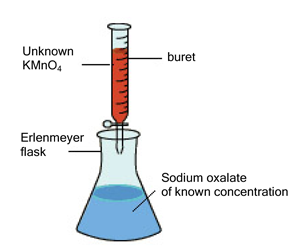# Volumetric Analysis

-Introduction

-Calculate Concentration Oxalic Acid

-Preparation of a Starndard Solution

-Evaluation Question: Virtual Lab

Experiment 4 Online Tutorial >> Volumetric Analysis: Potassium Permanganate Titration >> Introduction

 Next >

# Experiment 4 - Volumetric Analysis: Potassium Permanganate Titration

## Introduction

In this experiment, your goal is to determine the concentration of unknown KMnO4 using sodium oxalate of known concentration.

The experimental setup and strategy are shown below.## Step 1: Preparing a Sodium oxalate of known concentration

What concentration do I want?
Determine the concentration of the oxalic acid for 25.00 mL of it to react completely with 25 mL of 0.01 M KMnO4 solution using the Calculate Concentration Oxalic Acid tutor on page 2.

How do I make the solution?
See Canvas EXP 4 Module on Making a Standard Solution.

What glassware do I use?
See Canvas EXP 4 module on Significant Figures & Volumetric Analysis.

## Step 2: Performing the Titration

Add titrant until solution turns constant pale pink colour.

Why does it turn pink?
Titration of KMnO4 (purple) into the oxalate solution (colourless), produces colourless Mn2+ until the end point. At the end point, the oxalate solution will be exhausted and the purple solution colour will be permanent.

How do I interpret the results?
When performing volumetric analysis calculations, consider how you will calculate the amount (number of moles) of KMnO4 in solution. This experiment uses a known amount of sodium oxalate. From this amount, consider how you can determine the concentration of KMnO4. Remember to consider the stoichiometry of this reaction in your calculations.

2MnO4- + 6H+ + 5H2C2O4 --> 2Mn2+ + 8H2O + 10CO2

Practice this reaction in the Virtual Lab
Perform a virtual titration of this problem using the ChemCollective Virtual Laboratory on page 4 of this tutorial.

The following pages contain tutors to assist you in the calculations required for the above steps. Please click next to continue to the tutors.

 Next >